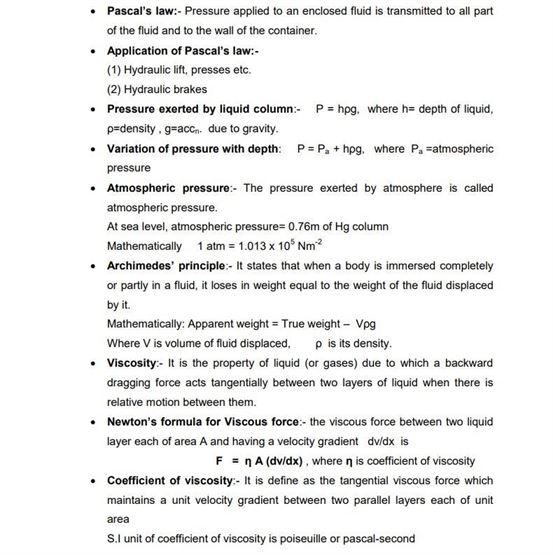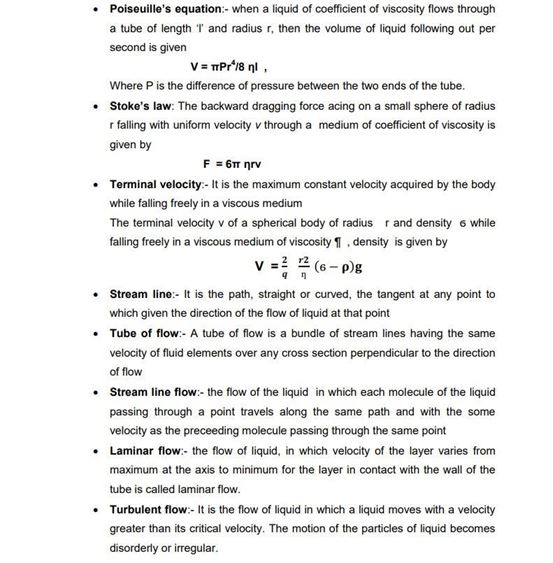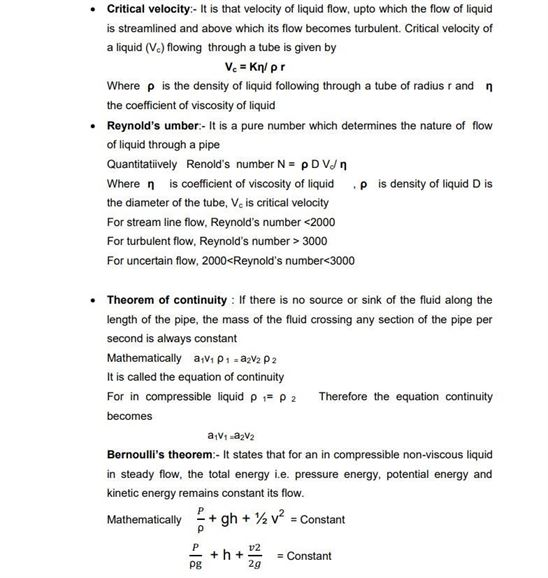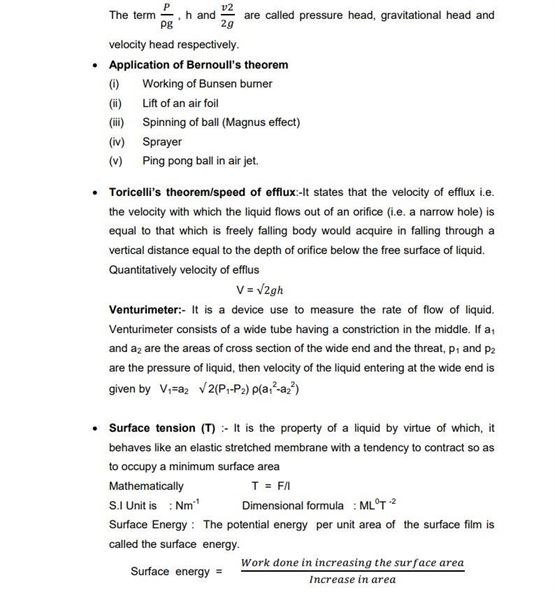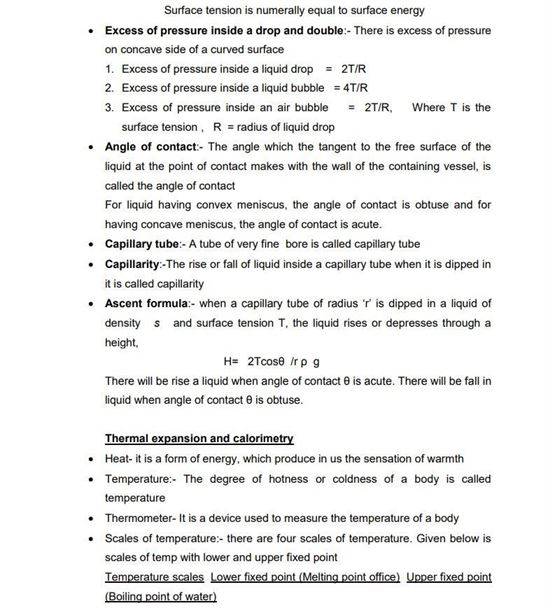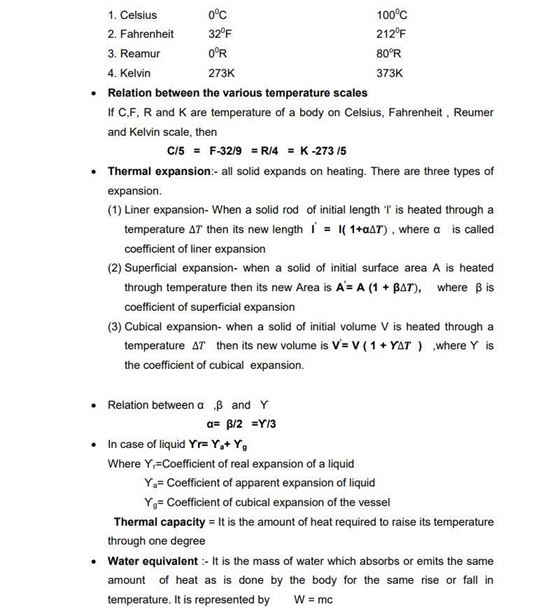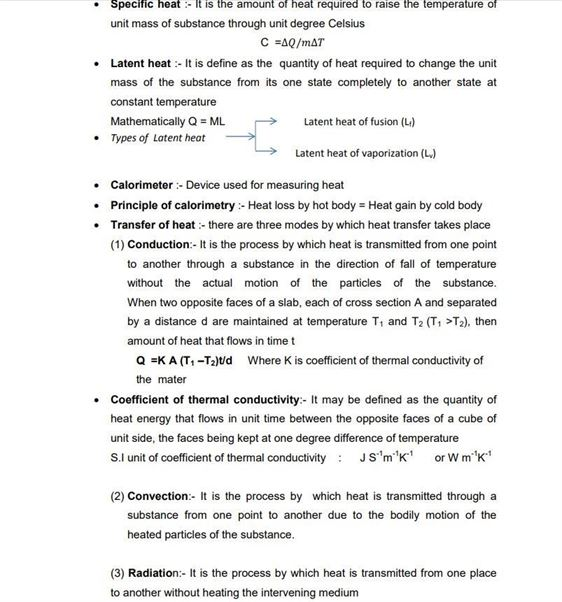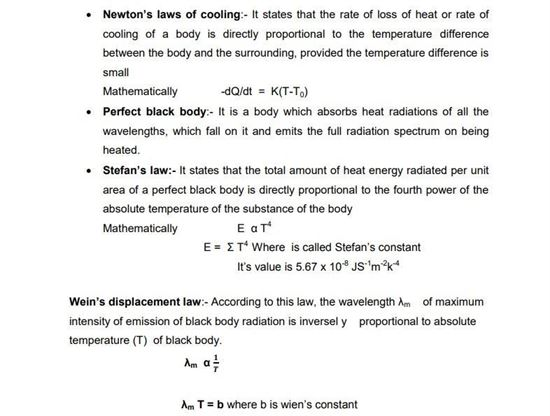# Physics Revision Notes for Class 11 Chapter 10 Mechanical Properties of Fluids

Here students can find the class 11 physics revision notes Chapter 10 Mechanical Properties Of Fluids. Students will find the definition of all the important topics in physics such as the Archimedes principle, laws of floatation, the equation of continuity, Bernoulli’s theorem etc.

• Archimedes’ principle states that the buoyant force applied to an object is equal to the weight of the fluid the object displaces
• According to Bernoulli’s theorem in physics, whenever there is an increase in the speed of the liquid, there is a simultaneous decrease in the potential energy of the fluid or we can say that there is a decrease in the pressure of the fluid
• The continuity equation deals with changes in the area of cross-sections of passages which fluids flow through. Laminar flow is flow of fluids that doesn’t depend on time, ideal fluid flow

They will also see the definition of Magnus effect, critical velocity, Reynold’s number etc. along with that, they will be exposed to tables showing the viscosities of fluids, Torricelli’s theorem. These notes will be very helpful to students who can find all the important concepts related to this chapter.

Find the class 11 physics revision notes Chapter 10 Mechanical Properties of Fluids here.